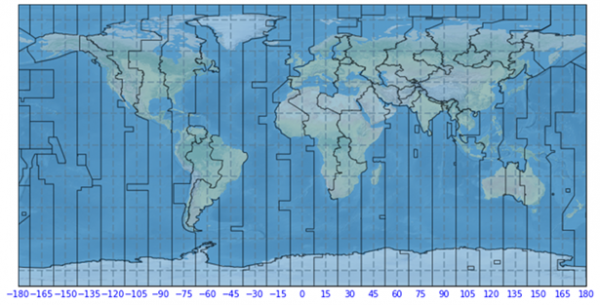# How to plot Time Zones in a map in Matplotlib

Let us consider that we have some data in which we have to deal with the actual time. To plot the time-zones on the map, we can use the ‘cartopy’ or ‘metPy’ package in Python. However, we can install ‘cartopy’ package in the Anaconda environment using the commands,

conda install -c conda-forge cartopy

Or

conda install -c conda-forge metpy

Now, let’s see how to plot time zones in a map using Matplotlib.

## Example

import numpy as np
import cartopy.crs as ccrs
import matplotlib.animation as animation
import matplotlib.pyplot as plt

#Defining the plot size and axes
plt.figure(figsize=(10, 9))
ax = plt.axes(projection=ccrs.PlateCarree())

#Apply the color for the natural Earth sourced from cartopy package
ax.stock_img()
ax.coastlines(zorder=1)

#Reading the timezone using ShapelyFeature of Cartopy
ccrs.PlateCarree(), edgecolor='black')

# Plot the feature on the map
gl = ax.gridlines(crs=ccrs.PlateCarree(), draw_labels=True,
linewidth=2, color='gray', alpha=0.5, linestyle='--')

#Apply the gridlines to separate the timezones
gl = ax.gridlines(crs=ccrs.PlateCarree(), draw_labels=True,
linewidth=2, color='black', alpha=0.5, linestyle='--')

#Turn off all the axes excluding bottom
gl.xlabels_top = False
gl.ylabels_left = False
gl.ylabels_right = False

#Apply fixed locations with the tickable objects
gl.xlocator = mticker.FixedLocator(np.linspace(-180, 180, 25))
gl.xlabel_style = {'size': 10, 'color': 'blue'}
ax.set_title('Global Time Zones', size=15, color='g')

#Display the plots
plt.show()

## Output

Running the above code snippet will generate the output as,